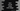# Dart program to concat string with integer## Introduction :

In this tutorial, we will learn how to concatenate one string with an integer. For example, if one integer 25 is given, we will concatenate with one string, say degree to create the final string 25 degree.

### Method 1: By converting the integer to a string:

We can convert the integer to string using toString() method and we can append that value to any other string using a plus sign.

``````void main() {
String givenStr = 'Degrees';
int value = 25;

String finalStr = value.toString() + ' ' + givenStr;
print(finalStr);
}``````

It will print :

``25 Degrees``

### Method 2: Using string interpolation :

String interpolation is another easy way to do it :

``````void main() {
String givenStr = 'Degrees';
int value = 25;

String finalStr = '\$value \$givenStr';
print(finalStr);
}``````

It will print the same output as the above example.